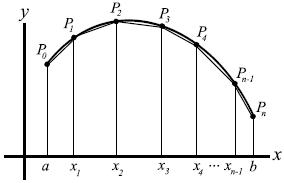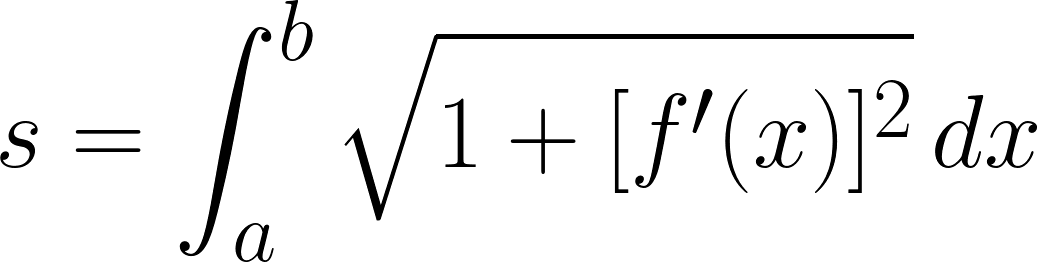### 6.E. Arc Length

Definite integrals can be used to find the arc lengths of curves. An arc (a segment of a curve) is approximated by straight line segments whose lengths are given by the distance equation.Arc LengthEssential Questions
• How can the arc length of a smooth curve be found?
Skills
• Find the arc length of a smooth curve.
Resources

6.E. Videos

#### Arc Length

The gadget spec URL could not be found